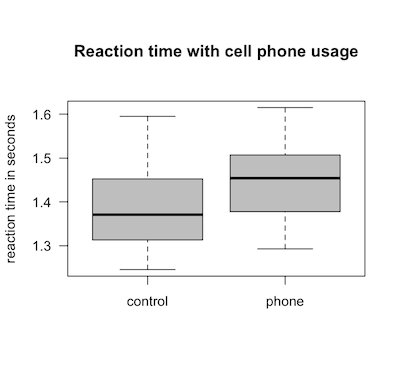## Sunday, June 06, 2010

### Using R for Introductory Statistics 3.2

...continuing my sloth-like progress through John Verzani's Using R for Introductory Statistics. Previous installments: Chapters 1 and 2 and 3.1.

### Comparing independent samples

Boxplots provide a visual comparison between two or more distributions. For problem 3.8, we're asked to compare the reaction times of cell phone users verses a control group, to test the theory that using a cell phone while driving is a bad idea. Comparing the centers and spreads can be done with the following boxplot.

```boxplot(time ~ control, reaction.time, names=c('control', 'phone'),
col='gray',
ylab='reaction time in seconds',
main='Reaction time with cell phone usage')
```The tilde operator, ~, is used to define a model formula, which is something I aspire to understand someday but currently am clueless about.

Looking at the same data as a density plot might give a better picture of each distribution.

```plot(density(reaction.time\$time[reaction.time\$control=='T']),
main="Reaction time with cell phone usage",
xlab="reaction time in seconds")
lines(density(reaction.time\$time[reaction.time\$control=='C']), lty=2)
```Still, boxplots are nice because they give you a sense of the center, range, dispersion, and skew of a sample in a compact and comparable form. Plus, you can plot several boxplots side-by-side.

```boxplot(morley\$Speed ~ morley\$Expt,
col='light grey', xlab='Experiment #',
ylab="speed (km/s minus 299,000)",
main="Michelson–Morley experiment")
mtext("speed of light data")
abline(h=sol, col='red')
```Problem 3.11 uses data from the 1887 Michelson-Morley experiments attempting to find variations in the speed of light due to earth's motion through the aether, believed at the time to be the medium through which light waves traveled. The correct value for the speed of light is shown in red.

And finally, whadya know, this stuff came in handy for some (probably not very rigorous) performance analysis.